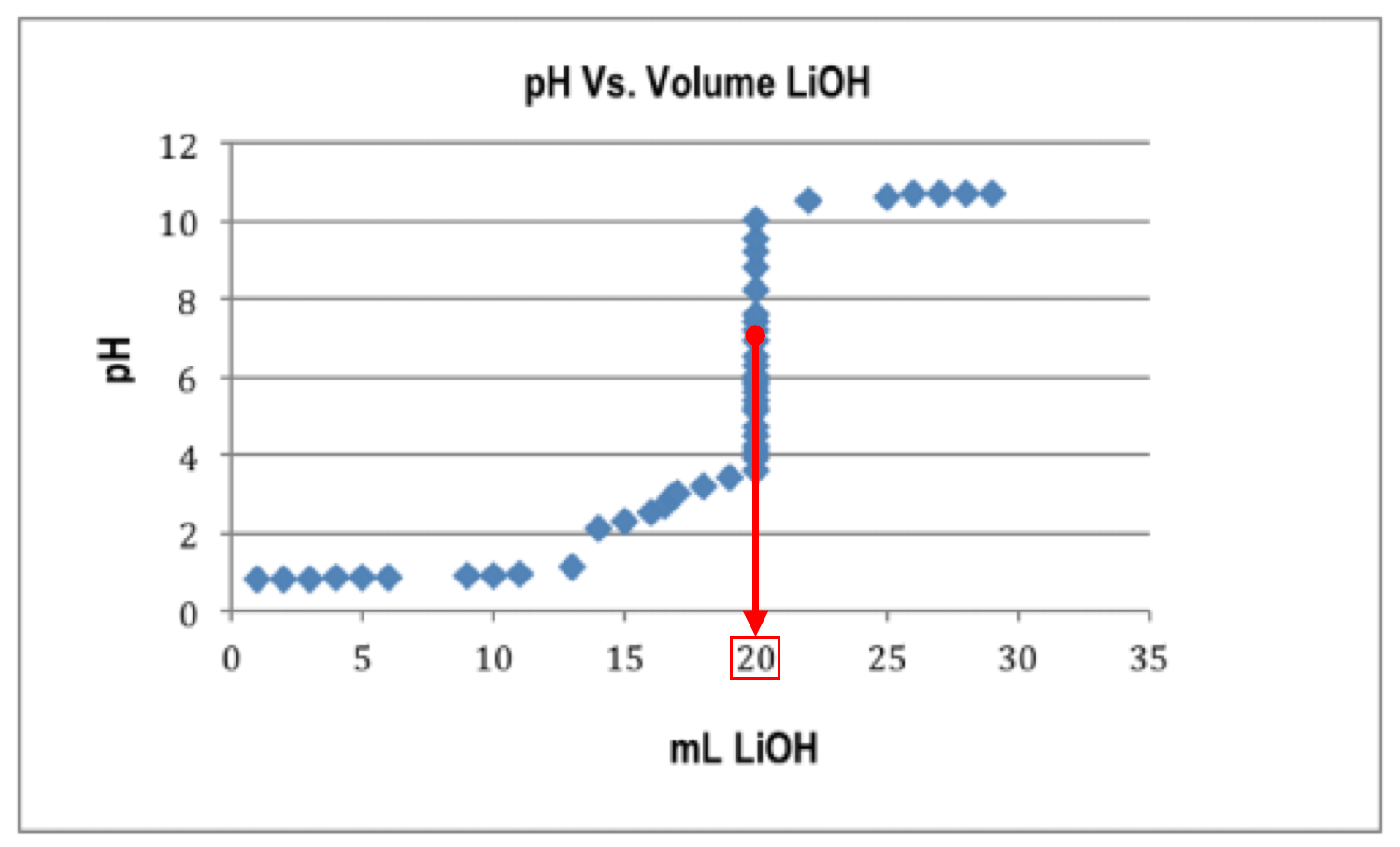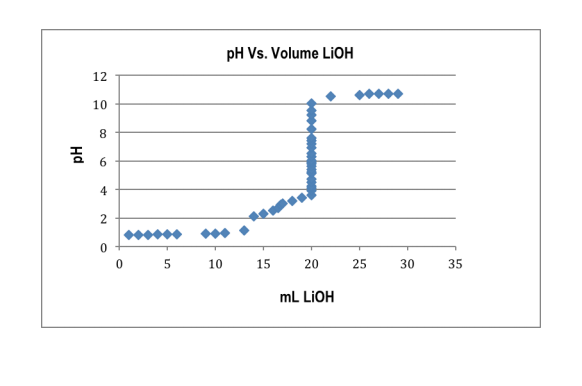# Problem: Consider the given graph. If the given graph shows the titration of 23.00 mL of an HNO3 solution of an unknown concentration with a 0.135 M LiOH solution, what is the molarity of the acid solution?

###### FREE Expert Solution

We’re being asked to calculate the molarity of HNO3 (strong acid) from the given titration data with LiOH (strong base)

Recall that in the titration of a strong acid and strong base, the equivalence point occurs at pH 7. From the titration curve, we can determine the volume of LiOH:This means that 23.00 mL of HNO3 required 20.00 mL of 0.135 M LiOH to reach the equivalence point.###### Problem Details

Consider the given graph. If the given graph shows the titration of 23.00 mL of an HNO3 solution of an unknown concentration with a 0.135 M LiOH solution, what is the molarity of the acid solution?